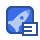# Python经典练习题

4星（超过85%的资源）2795收藏

year= int(raw input( year: \n) month=int(raw input[' month:n n ) day=int(raw input(' day n) months=0,31,59,90,120151,181,212,243,273,304,334) if 0<= month < 12. sum=months[month-1 print data error sum + day 0 if (year%400==0)or (year %4==0)and year % 1 =0) leap =1 if (leap ==1)and(month >2) sum +=1 print it is the %dth day. 9 sum 【程序5】 题日:输入三个整数xy,z,请把这三个数由小到大输出。 1程序分析:我们想办沄把最小的数放到x上,先将x与y进行比较,如果xy则将x与y 的值进行交换, 然后再用ⅹ与z进行比较,如果xz则将ⅹ与z的值进行交换,这样能使κ最 2程序源代码: for i in range 3): x=int(raw input(integer \n) . append(x) 【程序6】 趟目:用*号输出字斗C的图案 1程序分析:可先用*号在纸上写出字母C,再分行输出。 2.程序源代码 print 'Hello Python warld !\n printF10 for i in range (5) print rint print*\n*6 【程序7】 题目:输出特殊图案,请在c环境中运行,看一看, Very beautifu! 1程序分析:字符共有256个。不同字符,图形不一样。 2程序源代码 a=176 h=219 print chr(b), chra), chr(a), chr(a), chr(b) print chr(a), chr(b), chr(a), chr(b), chr(a) print chr(a), chr(a), chr(b), chr(a), chr(a) print chrfa), chr(b), chra), chr(b), chr(a print chr(b), chr(a), chr(a), chr(a), chr(b) 【程序8】 题目:输出9°9口诀。 1程序分析:分行与列考虑,共9行9列,i控制行,j控制列。 2程序源代码: include stdio. h main() int i,j, result: print("\n") for(i=1<10;++) [for(j=1小j<10)4+) result=i j; prnt("%d*%=%3d",j, result/*-3d表示左对齐,占3位 printf(("n");/*每一行后换行* for i in range(1, 10) for j in range (1, 10): result=i*j print‰d*‰d=%3d”‰ (i,j, result) n uId 【程序9】 题日:要求输出国际象棋棋盘。 1程序分析:用i控制行,j来控制列,根据的和的变化来控制输出黑方格,还是白方格。 2程序源代码: include stdio. h" maIn 1() Int for(=0i<8+) if(+)%2==0 printf("%Gcc",219,219); els printf(") printf("\n"); Import sys or I in range (8): for j in range (8) f+j%2=0: sys.sto te(chr(219) sys. stdout. write(chr(219)) else stdout. write[") print 【程序10】 趣目:打印楼梯,同时在楼梯上方打印两个笑脸 1程序分析:用i控制行,j来控制列,j根摇i的变化来控制输出黑方格的个数。 2程序源代码: sys. stdout. write(chr(1) sys. stdout. write(chr(1) print for」 in range(1,) sys. stdout write (chr(219)) sYs. stdout. write(chr(219) u uud 【程序11】 题目:古典问题:有对兔了,从出生后第3个月起每个月都生对兔了,小兔了长到第三 个月 后每个月又生一对兔子,假如兔子都不死,问每个月的兔子总数为多少? 1程序分析:兔子的规律为数列1,1,2,35,8,13,21 2.程序源代码 main long f1, f2: int i: f1=f2=1 for(i=1i<=20;++) {prnt"%12d‰12d",斤1,f2); f%2==0) printf("n");/控制输出,每行四个* f1-f1+f2;/*前两个月加起来赋值给第三个月* 2-f1;/前两个月加起来赋值给第三个月* f1=1 f2=1 for i in range (1, 21) print %12d %12d'%(f1, f2) if(i%2)=0: rint f1=f1+f2 f2=f1+f2 【程序12】 题目:判断101-200之间有多少个素数,并输出所有素数。 1程序分析:判断素数的方法:用一个数分別去除2到sqrt(这个数),如果能被整除, 则表明此数不是素数,反之是素数。 2程序源代码 h=0 leap= 1 from math import sqrt from sys import stdout for m in range (101, 201): k= int(sqrt(m+ 1)) for i in range (2, k+ 1) if m %i==0 leap=0 rea if le print %-4d% h=1 ifh%10=0 eap prnt' The total is‰%h 【程序13】 题目:打印出所有的“水仙花数”,所谓“水仙花数”是指一个三位数,其各位数字立方和 等于该数 本身。例如:153是一个“水仙花数”,因为153=1的三次方+5的三次方+3的三次 1程序分析:利用for循环控制100-999个数,每个数分解出个位,十位,百位 2程序源代码 for n in range( 100, 100 i=n/100 j=n/10%10 k=n%10 fi*100+j*10+k==i+j*2+k*3 print"‰-5d"%n 【程序14】 题目:将一个正整数分解质因数。例如:输入90打印出90=2*3*3*5。 程序分析:对n进行分解质因数,应先找到一个最小的质数k,然后按卜述步骤完成 (1)如果这个质数恰等于n,则说明分解质因数的过程已经结束,打印出即可。 (2)如果n<k,但n能被k整除,则应打印出k的值,并用n除以k的商作为新的正整数你 重复执行第一步。 (3如果n不能被k整除,则用k+1作为k的值,重复执行第步 2.程序源代码: from sys import stdout n= int(raw input("input number: \ n")) t"n=%"%n for i in range (2, n +1) while n l= i if n %i==0 tout. write(str( stdout. write( 0=h e se print"‰d"%n 【程序15】 题日:利用条件运算符的嵌套来完成此题:学习成绩>=90分的同学用A表示,60-89分之间 的用B表示, 60分以下的用C表小。 1程序分析:(a>b)?a:这是条件运算符的基本例子。 2程序源代码: 不支持这个运算符 score= int(raw input( input score: n ) if score >=90: grade =A elif score >=60 grade='B else. grade='C print"%d belongs to %s%(score grade) 【程序17】 题目:输入一行字符,分别统计出其中英文字母、空格、数字和其它字符的个数。 1程序分析:利用whe语句条件为输入的字符不为"n 2稈序源代码 mport string s=raw_ input(input a string: \n y letters=0 space =0 digit =0 others =o for c in s if c. isalpha0 letters+=1 elIf CIs spacel elif c isdigit(: digit +=1 s others +=1 print 'char=%d, space =%d, digit=%d, others=%d%(letters, space, digit, others) 题目:求s=a+a+aa+a3aa+aa.a的值,其中a是一个数字。例如2+22+22222222 共有5个数相加),几个数相加有键盘控制 1程序分析:关键是计算出每一项的值。 2程序源代码 Tn=o n=int(raw input('n =:\n) a=int(raw inputa=:n)) for count in range(n) In=Tn + a =a*10 Sn append(Tn) print Tn Sn=reduce (lambda x,y: x+y, Sn rint sn 【程序19】 题目:一个数如果恰好等于它的因子之和,这个数就称为“完数”例如6=1+2+3编程 找出1000以内的所有完数。 1.程序分析:请参照程序<-上页程序14. 2程序源代码: from sys import stdout forj in range( 2, 1001) k=[ n=-1 s for i in range (1, 行fj%i==0 1 S-=I kappend o if s==O pr for i in range(n) stdout. write(k[i) tout. write print k[n] 【程序20】 题目:一球从100米高度自由落下,每次落地后反跳回原高度的一半;再落下,求它在 第10次落地时,共经过多少米?第10次反弹多高? 1程序分析:见下面注释 2程序源代码 sn=100.0 Hn= Sn/2 for n in range (2, 11) Sn +=2* Hn Hn/=2 print Total of road is %f% Sn print The tenth is %f meter%Hn 【程序21】 题目:猴子吃桃河题:猴子第一天摘下若干个桃子,当即吃了一半,还不瘾,又多吃了一个 第二天早上又将剩下的桃子吃掉一半,又多吃了一个。以后每天早上都吃了前一天剩 的一半零一个。到第10天早上想再吃时,见只剩下一个桃子了。求第一天共摘了多 1程序分析:采取逆向思维的方法,从后往前推断。 2程序源代码: x2=1 for day in range (9, 0, -1) 1=(x2+1) 2=X1 print xl 【程序22】 趟目:两个乒乓球队进行比赛,各出三人。甲队为a,bc三人,乙队为xy2z三人。已抽签决 比赛名单。有人向队员打听比赛的名单。a说他不和ⅹ比,c说他不和x,z比,请编程 序找出 三队赛手的名单。 1程序分析:判断素数的方法:用一个数分别去陰2到sqrt(这个数),如果能被整除, 则表明此数不是素数,反之是素数 2程序源代码: for i in range(ord(x),ard(z)+1)

...展开详情

2018-04-10qq_40138490 被骗了，就是百度 https://wenku.baidu.com/view/f364fb2caeaad1f347933f7c.html 一摸一样
2017-12-21

2017-11-10

2017-10-18

2017-10-10

2017-09-30

2017-09-27

2017-09-11

2017-05-19

2016-04-19

• 15
资源
• 8
粉丝
•等级Python经典练习题 42积分/C币 立即下载
1/4242积分/C币 立即下载